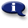# .

## Syllabus Information

Fall 2022
Mar 28,2023Use this page to maintain syllabus information, learning objectives, required materials, and technical requirements for the course.

Syllabus Information
MTH 060 - Beginning Algebra
Associated Term: Fall 2022
Learning Objectives: Upon successful completion of this course, the student will be able to:
1. Maintain, use, and expand skills and concepts learned in previous mathematics courses. Perform operations with fractions and decimals. Use unit analysis to convert units and solve problems
2. Perform addition, subtraction, multiplication, and division of rational numbers
3. Use and apply the concepts and language of algebraic expressions. Use variables to construct algebraic expressions. Evaluate algebraic expressions and simplify expressions using order of operations
Simplify algebraic expressions by removing parentheses and combining like terms
4. Solve linear equations and inequalities. Solve linear equations and formulas algebraically. Solve linear inequalities and graph their solutions on a number line
5. Use algebra to solve application problems. Translate verbal models into algebraic expressions and/or equations. Solve problems. Solve problems involving simple interest, motion, and mixtures. Solve problems using ratios and proportions. Solve problems involving similar triangles. Solve geometry problems involving perimeter, area, and volume
6. Interpret information represented numerically and graphically, and recognize linear relationships represented verbally, numerically, graphically, and algebraically. Read and interpret information given in a table or graph. Locate points in a rectangular coordinate system and represent equations in two variables graphically. Identify the horizontal and vertical intercepts of the graph of an equation and interpret them in terms of an application. Identify the slope of a line and interpret it in terms of an application. Use the slope-intercept form of the equation of a line
7. Make appropriate and efficient use of a scientific calculator (Note: Students will be expected to demonstrate achievement of some objectives without the use of a calculator)
Required Materials:
Technical Requirements: